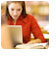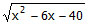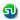### Inequalities of a quadratic function for real values of x. Inequality Problems. Ascent TANCET, CAT, PGSEM Correspondence course

 Home TANCET Classes GMAT Coaching Online GMAT Course CAT Classes TANCET Study Material
 TANCET '18 Classroom ProgramWeekend and weekday classes for TANCET MBA @ Chennai. At Nungambakkam and Velachery.Other Courses TANCET Correspondence Course CAT Classes & Courses GMAT Classes Chennai Online GMAT Course GRE Classes Chennai CBSE Math Online Tuition SAT Classes Other Links Ascent TANCET Toppers Testimonials CAT, TANCET Questions Careers @ Ascent Contact Us +91 44 4500 8484 +91 96000 48484 ascent@ascenteducation.com Postal Address Facebook / Twitter / Blog / Videos
You are here: Home  »  CAT, XAT, TANCET Prep Questions »  Inequalities »   Question 6

# Quantitative Reasoning : Inequalities

## Question

For what values of 'x' is the functiondefined in the real domain?
1. -10 < x < 4
2. 4 < x < 10
3. x does not lie between the closed interval 10 and 4
4. x does not lie between the open interval 4 and 10

Choice (4). x does not lie between the open interval 4 and 10

The functionis defined in the real domain only when x2  6x  40 > 0.

When x2  6x  40 is < 0, the function will be imaginary.

Now let us find out the range of values for which x2  6x  40 > 0.

Factorizing the quadratic expression, we get (x  10)(x + 4) > 0

This expression (x  10)(x + 4) will be greater than or equal to 0 when both (x  10) and (x + 4) are greater than or equal to 0 or when both (x - 10) and (x + 4) are less than or equal 0.

## Case 1:

When both (x  10) and (x + 4) are greater than or equal to 0.

x > 10 and x > - 4 => when x > 10 it will be greater than 4.

Therefore, it will suffice to say that x > 10

## Case 2:

When both (x - 10) and (x + 4) are less than or equal to 0.

i.e. x < 10 and x < -4 => when x < -4, it will less than 10.

Therefore, it will suffice to say that x < -4

Hence, the range in which the given function will be defined in the real domain will be when x does not lie between 4 and 10.

## CAT, XAT, TANCET Practice Questions and Answers : Listed Topicwise

 Number Theory Permutation Combination Probability Inequalities Geometry Mensuration Trigonometry Coordinate Geometry Percentages Profit Loss Ratio Proportion Mixtures Alligation Speed Time Distance Pipes Cisterns Interest Races Average, Mean AP, GP, HP Set Theory Clocks Calendars Algebra Function English Grammar General Awareness Data sufficiency TANCET Papers XAT PapersAdd to del.icio.usStumble Itdigg this